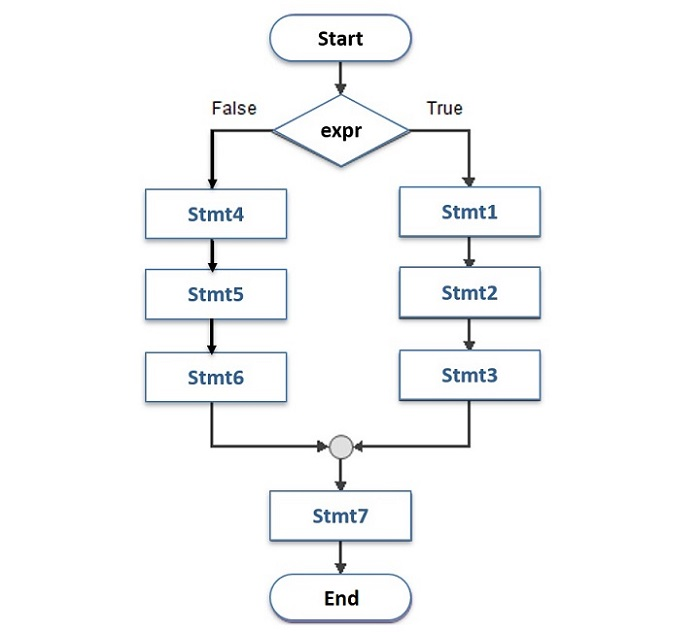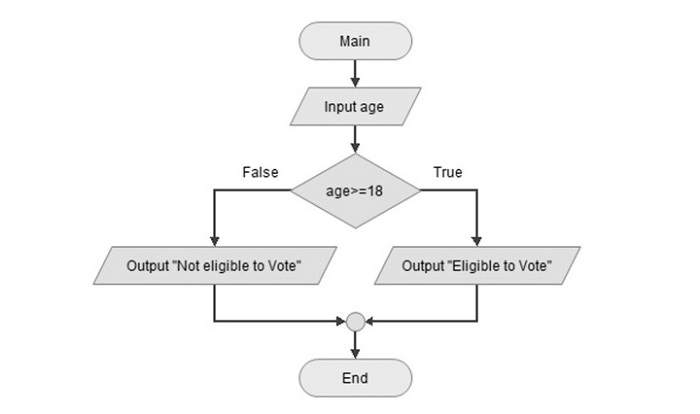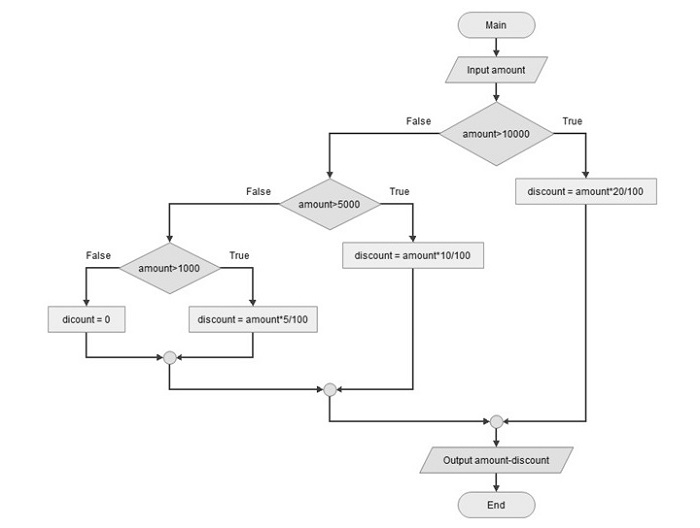# Python - The if-else Statement

Along with the if statement, else keyword can also be optionally used. It provides an alternate block of statements to be executed if the Boolean expression (in if statement) is not true. this flowchart shows how else block is used.If the expr is True, block of stmt1,2,3 is executed then the default flow continues with stmt7. However, the If expr is False, block stmt4,5,6 runs then the default flow continues.

### Syntax

Python implementation of the above flowchart is as follows −

```if expr==True:
stmt1
stmt2
stmt3
else:
stmt4
stmt5
stmt6
Stmt7
```

### Example

Let us understand the use of else clause with following example. The variable age can take different values. If the expression "age > 18" is true, message you are eligible to vote is displayed otherwise not eligible message should be displayed. Following flowchart illustrates this logic.Its Python implementation is simple.

```age=25
print ("age: ", age)
if age>=18:
print ("eligible to vote")
else:
print ("not eligible to vote")
```

To begin, set the integer variable "age" to 25.

Then use the if statement with "age>18" expression followed by ":" which starts a block; this will come in action if "age>=18" is true.

To provide else block, use "else:" the ensuing indented block containing message not eligible will be in action when "age>=18" is false.

On executing this code, you will get the following ouput

```age: 25
eligible to vote
```

To test the the else block, change the age to 12, and run the code again.

```age: 12
not eligible to vote
```

## Python â€“ elif Statement

The elif statement allows you to check multiple expressions for TRUE and execute a block of code as soon as one of the conditions evaluates to TRUE.

Similar to the else statement, the elif statement is optional. However, unlike else, for which there can be at the most one statement; there can be an arbitrary number of elif statements following an if.

### Syntax

```if expression1:
statement(s)
elif expression2:
statement(s)
elif expression3:
statement(s)
else:
statement(s)
```

### Example

Let us understand how elif works, with the help of following example.

The discount structure used in an earlier example is modified to different slabs of discount −

• 20% on amount exceeding 10000,

• 10% for amount between 5-10000,

• 5% if it is between 1 to 5000.

• no discount if amount<1000

The following flowchart illustrates these conditions −### Example

We can write a Python code for the above logic with if-else statements −

```amount = int(input('Enter amount: '))
if amount > 10000:
discount = amount * 20 / 100
else:
if amount > 5000:
discount = amount * 10 / 100
else:
if amount > 1000:
discount = amount * 5 / 100
else:
dicount = 0
print('amount: ',amount - discount)
```

While the code will work perfectly ok, if you look at the increasing level of indentation at each if and else statement, it will become difficult to manage if there are still more conditions.

The elif statement makes the code easy to read and comprehend.

Elif is short for "else if". It allows the logic to be arranged in a cascade of elif statements after the first if statement. If the first if statement evaluates to false, subsequent elif statements are evaluated one by one and comes out of the cascade if any one is satisfied.

Last in the cascade is the else block which will come in picture when all preceding if/elif conditions fail.

```amount = 800
print('amount = ',amount)
if amount > 10000:
discount = amount * 20 / 100
elif amount > 5000:
discount = amount * 10 / 100
elif amount > 1000:
discount = amount * 5 / 100
else:
discount=0

print('payable amount = ',amount - discount)
```

Set amount to test all possible conditions: 800, 2500, 7500 and 15000. The outputs will vary accordingly −

```amount: 800
payable amount = 800
amount: 2500
payable amount = 2375.0
amount: 7500
payable amount = 6750.0
amount: 15000
payable amount = 12000.0
```

## Nested If Statements

There may be a situation when you want to check for another condition after a condition resolves to true. In such a situation, you can use the nested if construct.

In a nested if construct, you can have an if...elif...else construct inside another if...elif...else construct.

### Syntax

The syntax of the nested if...elif...else construct will be like this −

```if expression1:
statement(s)
if expression2:
statement(s)
elif expression3:
statement(s)3
else
statement(s)
elif expression4:
statement(s)
else:
statement(s)
```

### Example

Now let's take a Python code to understand how it works −

```# !/usr/bin/python3
num=8
print ("num = ",num)
if num%2==0:
if num%3==0:
print ("Divisible by 3 and 2")
else:
print ("divisible by 2 not divisible by 3")
else:
if num%3==0:
print ("divisible by 3 not divisible by 2")
else:
print ("not Divisible by 2 not divisible by 3")
```

When the above code is executed, it produces the following output

```num = 8
divisible by 2 not divisible by 3
num = 15
divisible by 3 not divisible by 2
num = 12
Divisible by 3 and 2
num = 5
not Divisible by 2 not divisible by 3
```